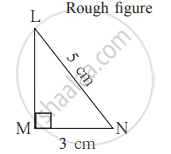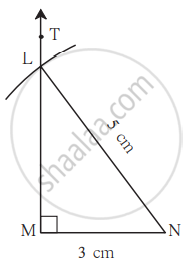# Construct a Right-angled Triangle Given the Hypotenuse and One Side

## Notes

### Construct a Right-angled Triangle Given the Hypotenuse and One Side:

1) Draw ∆LMN such that m∠LMN = 90°, hypotenuse = 5 cm, l(MN) = 3 cm.

Let us draw the rough figure using the given information.
As m∠LMN = 90°, we draw a right-angled triangle approximately and mark the right angle. Thus we show the given information in the rough figure.#### Steps:

1. As shown in the rough figure, draw the base seg MN of length 3 cm.
2. At point M of seg MN, draw ray MT to make an angle of 90° to seg MN.
3. Opening the compass to 5 cm and with the point at N, draw an arc to cut seg MT at L.
∆LMN is the required triangle.4. Note that a similar figure can be drawn on the other side of the base.
If you would like to contribute notes or other learning material, please submit them using the button below.

### Shaalaa.com

Drawing a Right Angled Triangle [00:04:00]
S
0%